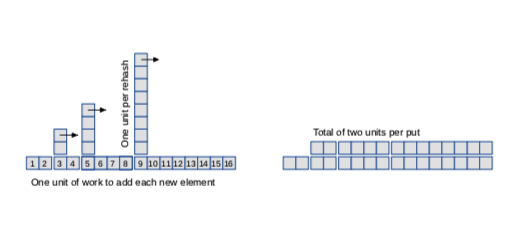# 11.2: Analyzing MyHashMap

$$\newcommand{\vecs}{\overset { \rightharpoonup} {\mathbf{#1}} }$$ $$\newcommand{\vecd}{\overset{-\!-\!\rightharpoonup}{\vphantom{a}\smash {#1}}}$$$$\newcommand{\id}{\mathrm{id}}$$ $$\newcommand{\Span}{\mathrm{span}}$$ $$\newcommand{\kernel}{\mathrm{null}\,}$$ $$\newcommand{\range}{\mathrm{range}\,}$$ $$\newcommand{\RealPart}{\mathrm{Re}}$$ $$\newcommand{\ImaginaryPart}{\mathrm{Im}}$$ $$\newcommand{\Argument}{\mathrm{Arg}}$$ $$\newcommand{\norm}{\| #1 \|}$$ $$\newcommand{\inner}{\langle #1, #2 \rangle}$$ $$\newcommand{\Span}{\mathrm{span}}$$ $$\newcommand{\id}{\mathrm{id}}$$ $$\newcommand{\Span}{\mathrm{span}}$$ $$\newcommand{\kernel}{\mathrm{null}\,}$$ $$\newcommand{\range}{\mathrm{range}\,}$$ $$\newcommand{\RealPart}{\mathrm{Re}}$$ $$\newcommand{\ImaginaryPart}{\mathrm{Im}}$$ $$\newcommand{\Argument}{\mathrm{Arg}}$$ $$\newcommand{\norm}{\| #1 \|}$$ $$\newcommand{\inner}{\langle #1, #2 \rangle}$$ $$\newcommand{\Span}{\mathrm{span}}$$$$\newcommand{\AA}{\unicode[.8,0]{x212B}}$$

If the number of entries in the biggest sub-map is proportional to $$\dfrac{n}{k}$$, and k grows in proportion to n, several of the core MyBetterMap methods become constant time:

public boolean containsKey(Object target) {
MyLinearMap<K, V> map = chooseMap(target);
return map.containsKey(target);
}

public V get(Object key) {
MyLinearMap<K, V> map = chooseMap(key);
return map.get(key);
}

public V remove(Object key) {
MyLinearMap<K, V> map = chooseMap(key);
return map.remove(key);
}


Each method hashes a key, which is constant time, and then invokes a method on a sub-map, which is constant time.

So far, so good. But the other core method, put, is a little harder to analyze. When we don’t have to rehash, it is constant time, but when we do, it’s linear. In that way, it’s similar to ArrayList.add, which we analyzed in Section 3.2.

For the same reason, MyHashMap.put turns out to be constant time if we average over a series of invocations. Again, the argument is based on amortized analysis (see Section 3.2).

Suppose the initial number of sub-maps, k, is 2, and the load factor is 1. Now let’s see how much work it takes to put a series of keys. As the basic “unit of work”, we’ll count the number of times we have to hash a key and add it to a sub-map.

The first time we call put it takes 1 unit of work. The second time also takes 1 unit. The third time we have to rehash, so it takes 2 units to rehash the existing keys and 1 unit to hash the new key.

Now the size of the hash table is 4, so the next time we call put, it takes 1 unit of work. But the next time we have to rehash, which takes 4 units to rehash the existing keys and 1 unit to hash the new key.

Figure $$\PageIndex{1}$$ shows the pattern, with the normal work of hashing a new key shown across the bottom and extra work of rehashing shown as a tower.Figure $$\PageIndex{1}$$: Representation of the work done to add elements to a hash table.

As the arrows suggest, if we knock down the towers, each one fills the space before the next tower. The result is a uniform height of 2 units, which shows that the average work per put is about 2 units. And that means that put is constant time on average.

This diagram also shows why it is important to double the number of sub-maps, k, when we rehash. If we only add to k instead of multiplying, the towers would be too close together and they would start piling up. And that would not be constant time.

This page titled 11.2: Analyzing MyHashMap is shared under a CC BY-NC-SA 3.0 license and was authored, remixed, and/or curated by Allen B. Downey (Green Tea Press) .